# Unit-2 raster scan graphics,line,circle and polygon algorithms

9 de Sep de 2020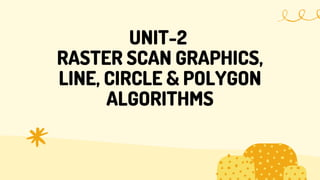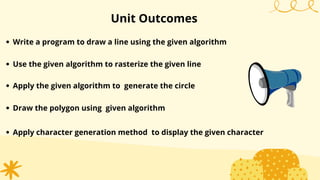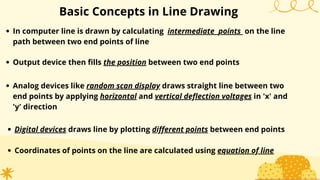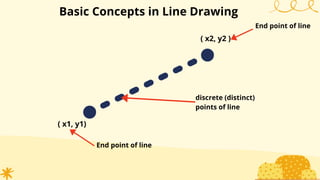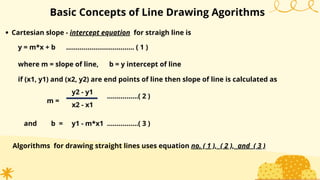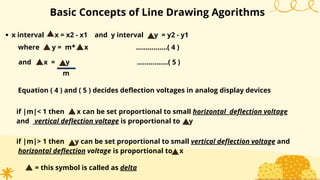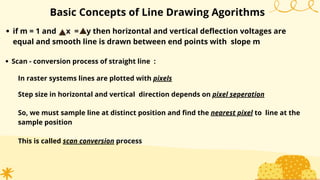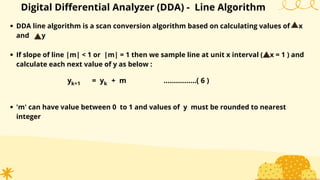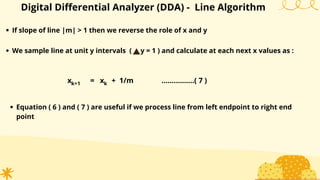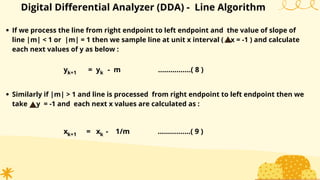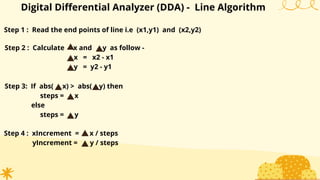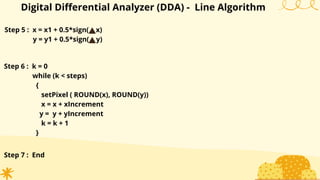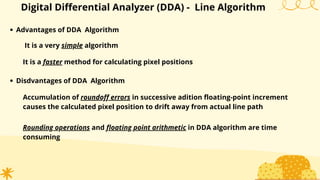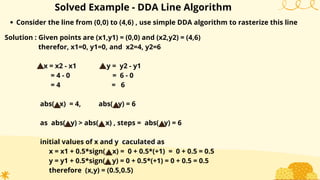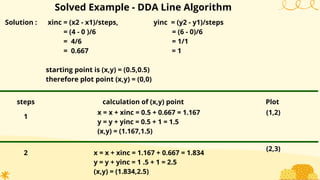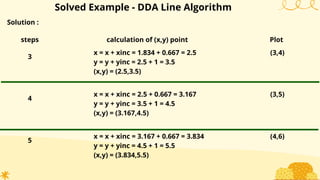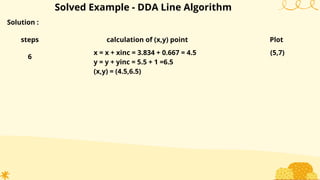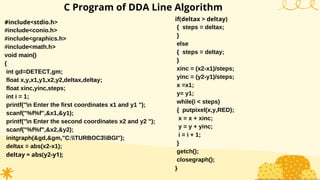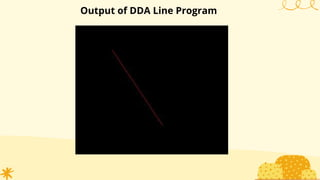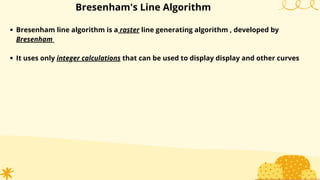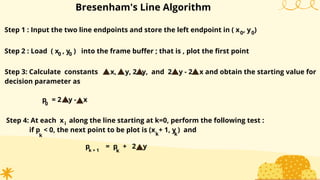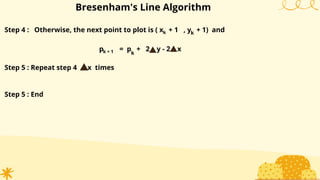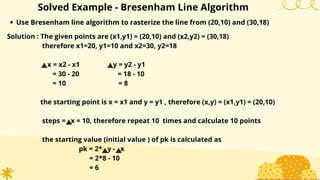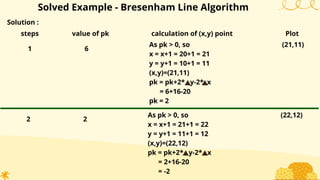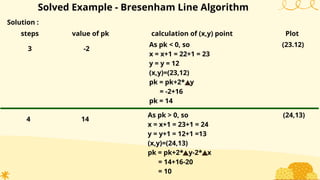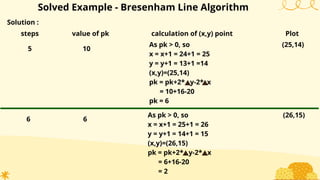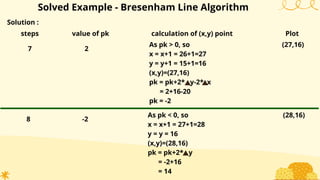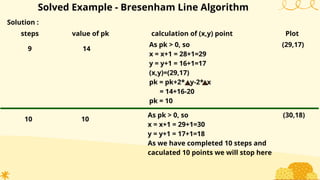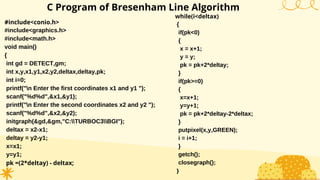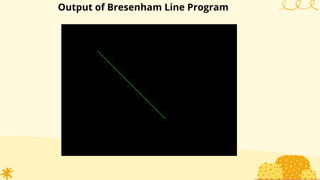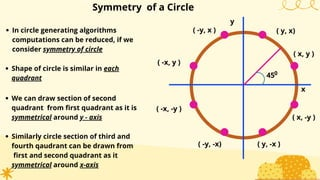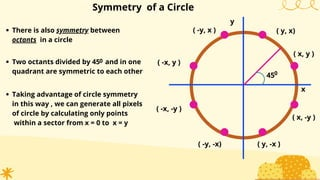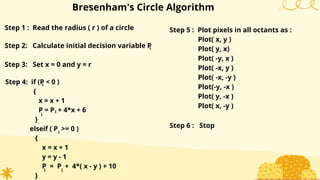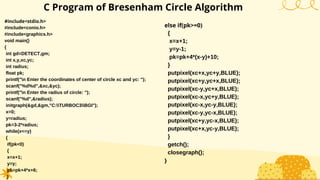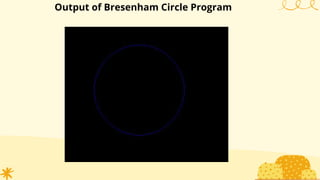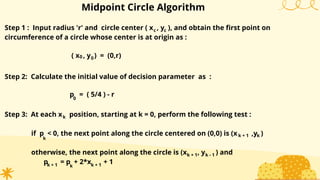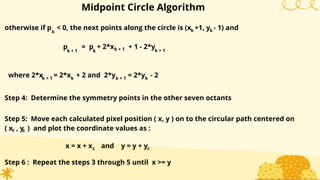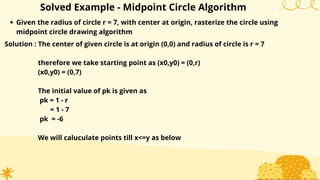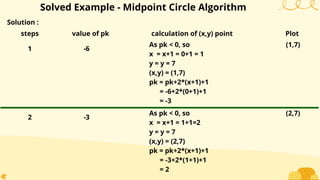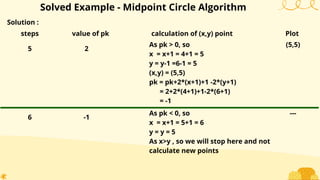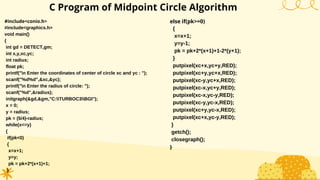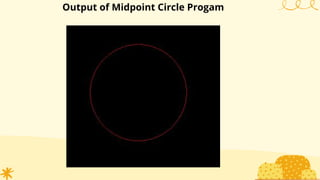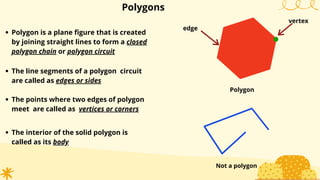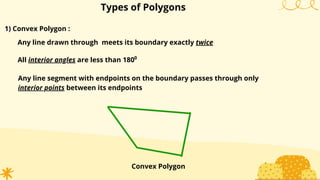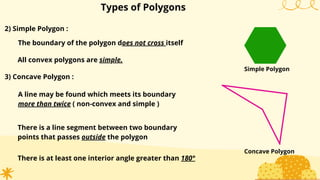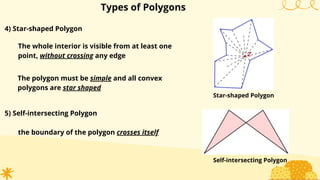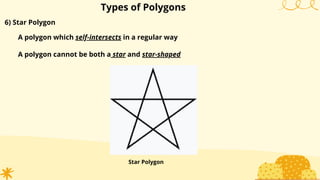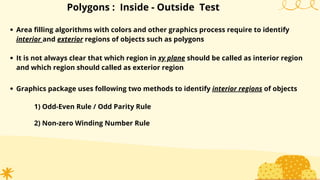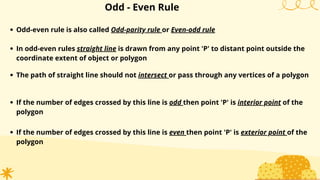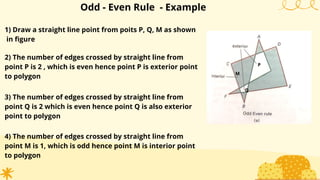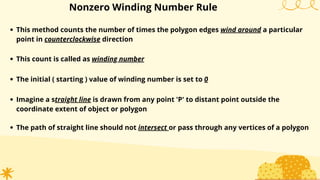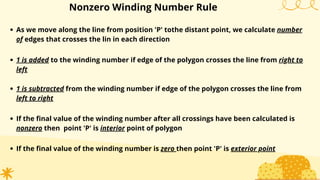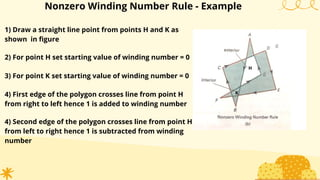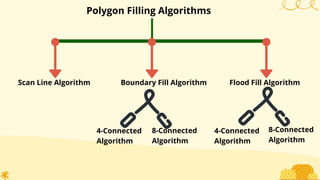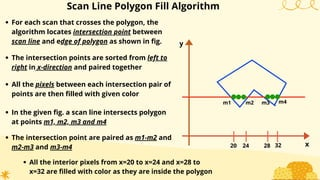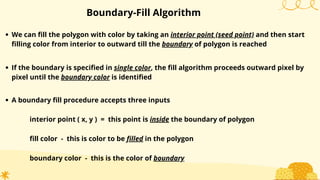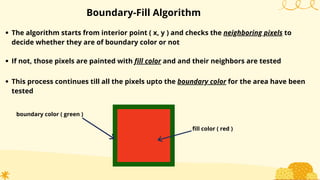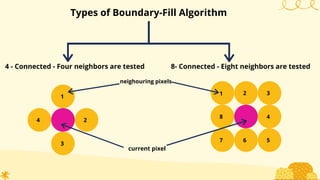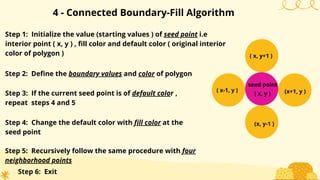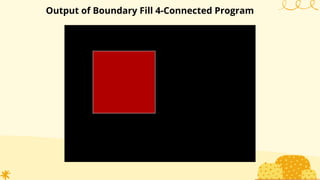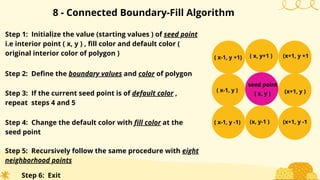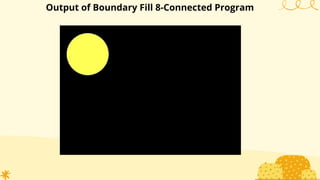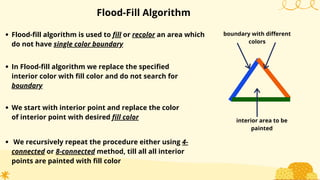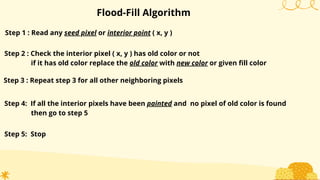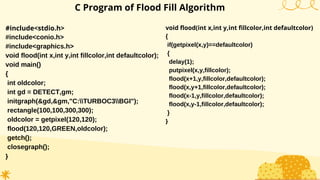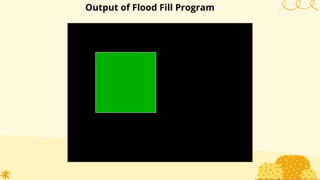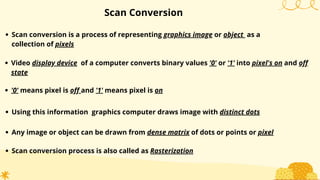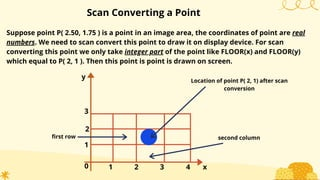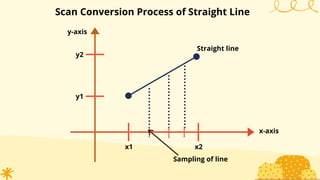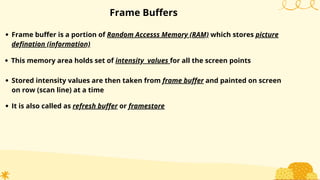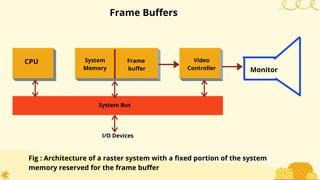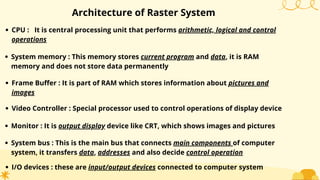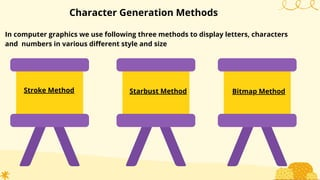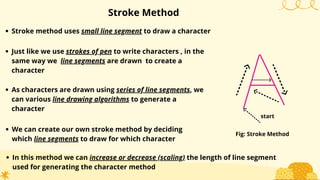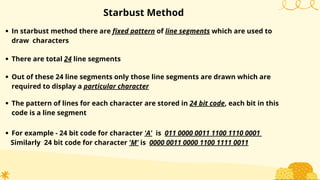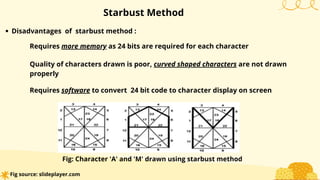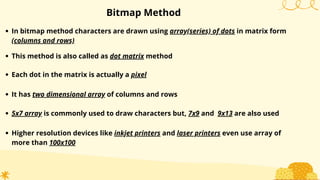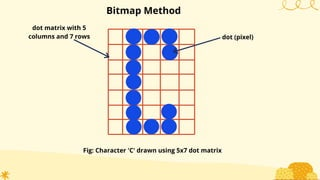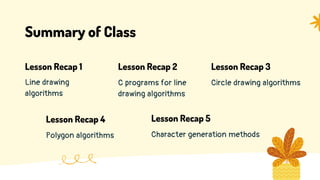1 de 87

### Unit-2 raster scan graphics,line,circle and polygon algorithms

• 1. COMPUTER GRAPHICS by Amol S. Gaikwad Lecturer Government Polytechnic Gadchiroli Maharashtra
• 2. UNIT-2 RASTER SCAN GRAPHICS, LINE, CIRCLE & POLYGON ALGORITHMS
• 3. Welcome! Are you excited for a fun learning session? Hi
• 4. Unit Outcomes Write a program to draw a line using the given algorithm Use the given algorithm to rasterize the given line Apply the given algorithm to generate the circle Draw the polygon using given algorithm Apply character generation method to display the given character
• 5. Basic Concepts in Line Drawing In computer line is drawn by calculating intermediate points on the line path between two end points of line Output device then fills the position between two end points Analog devices like random scan display draws straight line between two end points by applying horizontal and vertical deflection voltages in 'x' and 'y' direction Digital devices draws line by plotting different points between end points Coordinates of points on the line are calculated using equation of line
• 6. Basic Concepts in Line Drawing End point of line ( x1, y1) ( x2, y2 ) discrete (distinct) points of line End point of line
• 7. Basic Concepts of Line Drawing Agorithms Cartesian slope - intercept equation for straigh line is y = m*x + b ................................... ( 1 ) where m = slope of line, b = y intercept of line if (x1, y1) and (x2, y2) are end points of line then slope of line is calculated as m = y2 - y1 x2 - x1 ................( 2 ) and b = y1 - m*x1 ................( 3 ) Algorithms for drawing straight lines uses equation no. ( 1 ), ( 2 ), and ( 3 )
• 8. Basic Concepts of Line Drawing Agorithms x interval x = x2 - x1 and y interval y = y2 - y1 where y = m* x ................( 4 ) Equation ( 4 ) and ( 5 ) decides deflection voltages in analog display devices if |m|< 1 then x can be set proportional to small horizontal deflection voltage and vertical deflection voltage is proportional to y and x = y ................( 5 ) m if |m|> 1 then y can be set proportional to small vertical deflection voltage and horizontal deflection voltage is proportional to x = this symbol is called as delta
• 9. if m = 1 and x = y then horizontal and vertical deflection voltages are equal and smooth line is drawn between end points with slope m Basic Concepts of Line Drawing Agorithms Scan - conversion process of straight line : In raster systems lines are plotted with pixels Step size in horizontal and vertical direction depends on pixel seperation So, we must sample line at distinct position and find the nearest pixel to line at the sample position This is called scan conversion process
• 10. Digital Differential Analyzer (DDA) - Line Algorithm DDA line algorithm is a scan conversion algorithm based on calculating values of x and y If slope of line |m| < 1 or |m| = 1 then we sample line at unit x interval ( x = 1 ) and calculate each next value of y as below : y = y + m ................( 6 )k+1 k 'm' can have value between 0 to 1 and values of y must be rounded to nearest integer
• 11. Digital Differential Analyzer (DDA) - Line Algorithm If slope of line |m| > 1 then we reverse the role of x and y x = x + 1/m ................( 7 )k+1 k Equation ( 6 ) and ( 7 ) are useful if we process line from left endpoint to right end point We sample line at unit y intervals ( y = 1 ) and calculate at each next x values as :
• 12. Digital Differential Analyzer (DDA) - Line Algorithm If we process the line from right endpoint to left endpoint and the value of slope of line |m| < 1 or |m| = 1 then we sample line at unit x interval ( x = -1 ) and calculate each next values of y as below : y = y - m ................( 8 )k+1 k Similarly if |m| > 1 and line is processed from right endpoint to left endpoint then we take y = -1 and each next x values are calculated as : x = x - 1/m ................( 9 )k+1 k
• 13. Digital Differential Analyzer (DDA) - Line Algorithm Step 1 : Read the end points of line i.e (x1,y1) and (x2,y2) Step 2 : Calculate x and y as follow - x = x2 - x1 y = y2 - y1 Step 3: If abs( x) > abs( y) then steps = x else steps = y Step 4 : xIncrement = x / steps yIncrement = y / steps
• 14. Digital Differential Analyzer (DDA) - Line Algorithm Step 5 : x = x1 + 0.5*sign( x) y = y1 + 0.5*sign( y) Step 6 : k = 0 while (k < steps) { setPixel ( ROUND(x), ROUND(y)) x = x + xIncrement y = y + yIncrement k = k + 1 } Step 7 : End
• 15. Advantages of DDA Algorithm Digital Differential Analyzer (DDA) - Line Algorithm It is a very simple algorithm It is a faster method for calculating pixel positions Disdvantages of DDA Algorithm Accumulation of roundoff errors in successive adition floating-point increment causes the calculated pixel position to drift away from actual line path Rounding operations and floating point arithmetic in DDA algorithm are time consuming
• 16. Consider the line from (0,0) to (4,6) , use simple DDA algorithm to rasterize this line Solved Example - DDA Line Algorithm Solution : Given points are (x1,y1) = (0,0) and (x2,y2) = (4,6) therefor, x1=0, y1=0, and x2=4, y2=6 x = x2 - x1 y = y2 - y1 = 4 - 0 = 6 - 0 = 4 = 6 abs( x) = 4, abs( y) = 6 as abs( y) > abs( x) , steps = abs( y) = 6 initial values of x and y caculated as x = x1 + 0.5*sign( x) = 0 + 0.5*(+1) = 0 + 0.5 = 0.5 y = y1 + 0.5*sign( y) = 0 + 0.5*(+1) = 0 + 0.5 = 0.5 therefore (x,y) = (0.5,0.5)
• 17. Solution : xinc = (x2 - x1)/steps, yinc = (y2 - y1)/steps = (4 - 0 )/6 = (6 - 0)/6 = 4/6 = 1/1 = 0.667 = 1 starting point is (x,y) = (0.5,0.5) therefore plot point (x,y) = (0,0) Solved Example - DDA Line Algorithm steps calculation of (x,y) point Plot 1 x = x + xinc = 0.5 + 0.667 = 1.167 y = y + yinc = 0.5 + 1 = 1.5 (x,y) = (1.167,1.5) (1,2) 2 x = x + xinc = 1.167 + 0.667 = 1.834 y = y + yinc = 1 .5 + 1 = 2.5 (x,y) = (1.834,2.5) (2,3)
• 18. Solution : Solved Example - DDA Line Algorithm steps calculation of (x,y) point Plot 3 x = x + xinc = 1.834 + 0.667 = 2.5 y = y + yinc = 2.5 + 1 = 3.5 (x,y) = (2.5,3.5) (3,4) 4 x = x + xinc = 2.5 + 0.667 = 3.167 y = y + yinc = 3.5 + 1 = 4.5 (x,y) = (3.167,4.5) (3,5) 5 x = x + xinc = 3.167 + 0.667 = 3.834 y = y + yinc = 4.5 + 1 = 5.5 (x,y) = (3.834,5.5) (4,6)
• 19. Solution : Solved Example - DDA Line Algorithm steps calculation of (x,y) point Plot 6 x = x + xinc = 3.834 + 0.667 = 4.5 y = y + yinc = 5.5 + 1 =6.5 (x,y) = (4.5,6.5) (5,7)
• 20. C Program of DDA Line Algorithm #include<stdio.h> #include<conio.h> #include<graphics.h> #include<math.h> void main() { int gd=DETECT,gm; float x,y,x1,y1,x2,y2,deltax,deltay; float xinc,yinc,steps; int i = 1; printf("n Enter the first coordinates x1 and y1 "); scanf("%f%f",&x1,&y1); printf("n Enter the second coordinates x2 and y2 "); scanf("%f%f",&x2,&y2); initgraph(&gd,&gm,"C:TURBOC3BGI"); deltax = abs(x2-x1); deltay = abs(y2-y1); if(deltax > deltay) { steps = deltax; } else { steps = deltay; } xinc = (x2-x1)/steps; yinc = (y2-y1)/steps; x =x1; y= y1; while(i < steps) { putpixel(x,y,RED); x = x + xinc; y = y + yinc; i = i + 1; } getch(); closegraph(); }
• 21. Output of DDA Line Program
• 22. Bresenham's Line Algorithm Bresenham line algorithm is a raster line generating algorithm , developed by Bresenham It uses only integer calculations that can be used to display display and other curves
• 23. Bresenham's Line Algorithm Step 1 : Input the two line endpoints and store the left endpoint in ( x , y ) Step 2 : Load ( x , y ) into the frame buffer ; that is , plot the first point 0 0 0 0 Step 3: Calculate constants x, y, 2 y, and 2 y - 2 x and obtain the starting value for decision parameter as p = 2 y - x 0 Step 4: At each x along the line starting at k=0, perform the following test : if p < 0, the next point to be plot is (x + 1, y ) and i k k k p = p + 2 yk + 1 k
• 24. Bresenham's Line Algorithm Step 4 : Otherwise, the next point to plot is ( x + 1 , y + 1) and k k k + 1 k p = p + 2 y - 2 x Step 5 : Repeat step 4 x times Step 5 : End
• 25. Solved Example - Bresenham Line Algorithm Use Bresenham line algorithm to rasterize the line from (20,10) and (30,18) Solution : The given points are (x1,y1) = (20,10) and (x2,y2) = (30,18) therefore x1=20, y1=10 and x2=30, y2=18 x = x2 - x1 y = y2 - y1 = 30 - 20 = 18 - 10 = 10 = 8 the starting point is x = x1 and y = y1 , therefore (x,y) = (x1,y1) = (20,10) steps = x = 10, therefore repeat 10 times and calculate 10 points the starting value (initial value ) of pk is calculated as pk = 2* y - x = 2*8 - 10 = 6
• 26. Solution : Solved Example - Bresenham Line Algorithm steps calculation of (x,y) point Plot 1 As pk > 0, so x = x+1 = 20+1 = 21 y = y+1 = 10+1 = 11 (x,y)=(21,11) pk = pk+2* y-2* x = 6+16-20 pk = 2 (21,11) value of pk 6 2 As pk > 0, so x = x+1 = 21+1 = 22 y = y+1 = 11+1 = 12 (x,y)=(22,12) pk = pk+2* y-2* x = 2+16-20 = -2 (22,12) 2
• 27. Solution : Solved Example - Bresenham Line Algorithm steps calculation of (x,y) point Plot 3 As pk < 0, so x = x+1 = 22+1 = 23 y = y = 12 (x,y)=(23,12) pk = pk+2* y = -2+16 pk = 14 (23.12) value of pk -2 4 As pk > 0, so x = x+1 = 23+1 = 24 y = y+1 = 12+1 =13 (x,y)=(24,13) pk = pk+2* y-2* x = 14+16-20 = 10 (24,13) 14
• 28. Solution : Solved Example - Bresenham Line Algorithm steps calculation of (x,y) point Plot 5 As pk > 0, so x = x+1 = 24+1 = 25 y = y+1 = 13+1 =14 (x,y)=(25,14) pk = pk+2* y-2* x = 10+16-20 pk = 6 (25,14) value of pk 10 6 As pk > 0, so x = x+1 = 25+1 = 26 y = y+1 = 14+1 = 15 (x,y)=(26,15) pk = pk+2* y-2* x = 6+16-20 = 2 (26,15) 6
• 29. Solution : Solved Example - Bresenham Line Algorithm steps calculation of (x,y) point Plot 7 As pk > 0, so x = x+1 = 26+1=27 y = y+1 = 15+1=16 (x,y)=(27,16) pk = pk+2* y-2* x = 2+16-20 pk = -2 (27,16) value of pk 2 8 As pk < 0, so x = x+1 = 27+1=28 y = y = 16 (x,y)=(28,16) pk = pk+2* y = -2+16 = 14 (28,16) -2
• 30. Solution : Solved Example - Bresenham Line Algorithm steps calculation of (x,y) point Plot 9 As pk > 0, so x = x+1 = 28+1=29 y = y+1 = 16+1=17 (x,y)=(29,17) pk = pk+2* y-2* x = 14+16-20 pk = 10 (29,17) value of pk 14 10 As pk > 0, so x = x+1 = 29+1=30 y = y+1 = 17+1=18 As we have completed 10 steps and caculated 10 points we will stop here (30,18) 10
• 31. C Program of Bresenham Line Algorithm #include<conio.h> #include<graphics.h> #include<math.h> void main() { int gd = DETECT,gm; int x,y,x1,y1,x2,y2,deltax,deltay,pk; int i=0; printf("n Enter the first coordinates x1 and y1 "); scanf("%d%d",&x1,&y1); printf("n Enter the second coordinates x2 and y2 "); scanf("%d%d",&x2,&y2); initgraph(&gd,&gm,"C:TURBOC3BGI"); deltax = x2-x1; deltay = y2-y1; x=x1; y=y1; pk =(2*deltay) - deltax; while(i<deltax) { if(pk<0) { x = x+1; y = y; pk = pk+2*deltay; } if(pk>=0) { x=x+1; y=y+1; pk = pk+2*deltay-2*deltax; } putpixel(x,y,GREEN); i = i+1; } getch(); closegraph(); }
• 32. Output of Bresenham Line Program
• 33. Symmetry of a Circle x y 450 ( -y, x ) ( -x, y ) ( -x, -y ) ( -y, -x) ( y, -x ) ( x, -y ) ( x, y ) ( y, x)In circle generating algorithms computations can be reduced, if we consider symmetry of circle Shape of circle is similar in each quadrant We can draw section of second quadrant from first quadrant as it is symmetrical around y - axis Similarly circle section of third and fourth qaudrant can be drawn from first and second quadrant as it symmetrical around x-axis
• 34. Symmetry of a Circle x y 450 ( -y, x ) ( -x, y ) ( -x, -y ) ( -y, -x) ( y, -x ) ( x, -y ) ( x, y ) ( y, x)There is also symmetry between octants in a circle Two octants divided by 45 and in one quadrant are symmetric to each other Taking advantage of circle symmetry in this way , we can generate all pixels of circle by calculating only points within a sector from x = 0 to x = y 0
• 35. Bresenham's Circle Algorithm Step 1 : Read the radius ( r ) of a circle Step 2: Calculate initial decision variable Pi Step 3: Set x = 0 and y = r Step 4: if (P < 0 ) { x = x + 1 P = P + 4*x + 6 } elseif ( P >= 0 ) { x = x + 1 y = y - 1 P = P + 4*( x - y ) + 10 } i i i i ii Step 5 : Plot pixels in all octants as : Plot( x, y ) Plot( y, x) Plot( -y, x ) Plot( -x, y ) Plot( -x, -y ) Plot(-y, -x ) Plot( y, -x ) Plot( x, -y ) Step 6 : Stop
• 36. C Program of Bresenham Circle Algorithm #include<stdio.h> #include<conio.h> #include<graphics.h> void main() { int gd=DETECT,gm; int x,y,xc,yc; int radius; float pk; printf("n Enter the coordinates of center of circle xc and yc: "); scanf("%d%d",&xc,&yc); printf("n Enter the radius of circle: "); scanf("%d",&radius); initgraph(&gd,&gm,"C:TURBOC3BGI"); x=0; y=radius; pk=3-2*radius; while(x<=y) { if(pk<0) { x=x+1; y=y; pk=pk+4*x+6; } else if(pk>=0) { x=x+1; y=y-1; pk=pk+4*(x-y)+10; } putpixel(xc+x,yc+y,BLUE); putpixel(xc+y,yc+x,BLUE); putpixel(xc-y,yc+x,BLUE); putpixel(xc-x,yc+y,BLUE); putpixel(xc-x,yc-y,BLUE); putpixel(xc-y,yc-x,BLUE); putpixel(xc+y,yc-x,BLUE); putpixel(xc+x,yc-y,BLUE); } getch(); closegraph(); }
• 37. Output of Bresenham Circle Program
• 38. Midpoint Circle Algorithm Step 1 : Input radius 'r' and circle center ( x , y ), and obtain the first point on circumference of a circle whose center is at origin as : k c c ( x , y ) = (0,r)0 0 Step 2: Calculate the initial value of decision parameter as : p = ( 5/4 ) - r0 Step 3: At each x position, starting at k = 0, perform the following test : if p < 0, the next point along the circle centered on (0,0) is (x ,y ) otherwise, the next point along the circle is (x , y ) and p = p + 2*x + 1 k k k + 1 k + 1 k - 1 k + 1 k k + 1
• 39. Midpoint Circle Algorithm otherwise if p < 0, the next points along the circle is (x +1, y - 1) and p = p + 2*x + 1 - 2*y where 2*x = 2*x + 2 and 2*y = 2*y - 2 k c k + 1 k k k + 1 k k + 1 k + 1 k k + 1 k Step 4: Determine the symmetry points in the other seven octants Step 5: Move each calculated pixel position ( x, y ) on to the circular path centered on ( x , y ) and plot the coordinate values as : x = x + x and y = y + yc c c Step 6 : Repeat the steps 3 through 5 until x >= y
• 40. Solved Example - Midpoint Circle Algorithm Given the radius of circle r = 7, with center at origin, rasterize the circle using midpoint circle drawing algorithm Solution : The center of given circle is at origin (0,0) and radius of circle is r = 7 therefore we take starting point as (x0,y0) = (0,r) (x0,y0) = (0,7) The initial value of pk is given as pk = 1 - r = 1 - 7 pk = -6 We will caluculate points till x<=y as below
• 41. Solution : steps calculation of (x,y) point Plot 1 As pk < 0, so x = x+1 = 0+1 = 1 y = y = 7 (x,y) = (1,7) pk = pk+2*(x+1)+1 = -6+2*(0+1)+1 = -3 (1,7) value of pk -6 Solved Example - Midpoint Circle Algorithm 2 As pk < 0, so x = x+1 = 1+1=2 y = y = 7 (x,y) = (2,7) pk = pk+2*(x+1)+1 = -3+2*(1+1)+1 = 2 (2,7) -3
• 42. Solution : steps calculation of (x,y) point Plot 3 As pk > 0, so x = x+1 = 2+1=3 y = y-1 =7-1 = 6 (x,y) = (3,6) pk = pk+2*(x+1)+1 -2*(y+1) = 2+2*(2+1)+1-2*(7+1) = -7 (3,6) value of pk 2 Solved Example - Midpoint Circle Algorithm 4 As pk < 0, so x = x+1 = 3+1 = 4 y = y = 6 (x,y) = (4,6) pk = pk+2*(x+1)+1 = -7+2*(3+1)+1 = 2 (4,6) -7
• 43. Solution : steps calculation of (x,y) point Plot 5 As pk > 0, so x = x+1 = 4+1 = 5 y = y-1 =6-1 = 5 (x,y) = (5,5) pk = pk+2*(x+1)+1 -2*(y+1) = 2+2*(4+1)+1-2*(6+1) = -1 (5,5) value of pk 2 Solved Example - Midpoint Circle Algorithm 6 As pk < 0, so x = x+1 = 5+1 = 6 y = y = 5 As x>y , so we will stop here and not calculate new points --- -1
• 44. C Program of Midpoint Circle Algorithm #include<conio.h> #include<graphics.h> void main() { int gd = DETECT,gm; int x,y,xc,yc; int radius; float pk; printf("n Enter the coordinates of center of circle xc and yc : "); scanf("%d%d",&xc,&yc); printf("n Enter the radius of circle: "); scanf("%d",&radius); initgraph(&gd,&gm,"C:TURBOC3BGI"); x = 0; y = radius; pk = (5/4)-radius; while(x<=y) { if(pk<0) { x=x+1; y=y; pk = pk+2*(x+1)+1; } else if(pk>=0) { x=x+1; y=y-1; pk = pk+2*(x+1)+1-2*(y+1); } putpixel(xc+x,yc+y,RED); putpixel(xc+y,yc+x,RED); putpixel(xc-y,yc+x,RED); putpixel(xc-x,yc+y,RED); putpixel(xc-x,yc-y,RED); putpixel(xc-y,yc-x,RED); putpixel(xc+y,yc-x,RED); putpixel(xc+x,yc-y,RED); } getch(); closegraph(); }
• 45. Output of Midpoint Circle Progam
• 46. Polygon is a plane figure that is created by joining straight lines to form a closed polygon chain or polygon circuit Polygons The line segments of a polygon circuit are called as edges or sides The points where two edges of polygon meet are called as vertices or corners The interior of the solid polygon is called as its body Polygon Not a polygon edge vertex
• 47. Any line segment with endpoints on the boundary passes through only interior points between its endpoints Types of Polygons 1) Convex Polygon : Any line drawn through meets its boundary exactly twice All interior angles are less than 1800 Convex Polygon
• 48. Types of Polygons 2) Simple Polygon : The boundary of the polygon does not cross itself All convex polygons are simple. 3) Concave Polygon : A line may be found which meets its boundary more than twice ( non-convex and simple ) There is a line segment between two boundary points that passes outside the polygon There is at least one interior angle greater than 180° Simple Polygon Concave Polygon
• 49. Types of Polygons The whole interior is visible from at least one point, without crossing any edge 4) Star-shaped Polygon The polygon must be simple and all convex polygons are star shaped 5) Self-intersecting Polygon the boundary of the polygon crosses itself Star-shaped Polygon Self-intersecting Polygon
• 50. Types of Polygons 6) Star Polygon A polygon which self-intersects in a regular way A polygon cannot be both a star and star-shaped Star Polygon
• 51. Polygons : Inside - Outside Test Area filling algorithms with colors and other graphics process require to identify interior and exterior regions of objects such as polygons It is not always clear that which region in xy plane should be called as interior region and which region should called as exterior region Graphics package uses following two methods to identify interior regions of objects 1) Odd-Even Rule / Odd Parity Rule 2) Non-zero Winding Number Rule
• 52. Odd - Even Rule Odd-even rule is also called Odd-parity rule or Even-odd rule In odd-even rules straight line is drawn from any point 'P' to distant point outside the coordinate extent of object or polygon The path of straight line should not intersect or pass through any vertices of a polygon If the number of edges crossed by this line is odd then point 'P' is interior point of the polygon If the number of edges crossed by this line is even then point 'P' is exterior point of the polygon
• 53. Odd - Even Rule - Example 1) Draw a straight line point from poits P, Q, M as shown in figure P Q M 2) The number of edges crossed by straight line from point P is 2 , which is even hence point P is exterior point to polygon 3) The number of edges crossed by straight line from point Q is 2 which is even hence point Q is also exterior point to polygon 4) The number of edges crossed by straight line from point M is 1, which is odd hence point M is interior point to polygon
• 54. Nonzero Winding Number Rule This method counts the number of times the polygon edges wind around a particular point in counterclockwise direction This count is called as winding number The initial ( starting ) value of winding number is set to 0 Imagine a straight line is drawn from any point 'P' to distant point outside the coordinate extent of object or polygon The path of straight line should not intersect or pass through any vertices of a polygon
• 55. Nonzero Winding Number Rule As we move along the line from position 'P' tothe distant point, we calculate number of edges that crosses the lin in each direction 1 is added to the winding number if edge of the polygon crosses the line from right to left 1 is subtracted from the winding number if edge of the polygon crosses the line from left to right If the final value of the winding number after all crossings have been calculated is nonzero then point 'P' is interior point of polygon If the final value of the winding number is zero then point 'P' is exterior point
• 56. Nonzero Winding Number Rule - Example H K 1) Draw a straight line point from points H and K as shown in figure 2) For point H set starting value of winding number = 0 3) For point K set starting value of winding number = 0 4) First edge of the polygon crosses line from point H from right to left hence 1 is added to winding number 4) Second edge of the polygon crosses line from point H from left to right hence 1 is subtracted from winding number
• 57. Polygon Filling Algorithms Scan Line Algorithm Boundary Fill Algorithm Flood Fill Algorithm 4-Connected Algorithm 4-Connected Algorithm 8-Connected Algorithm 8-Connected Algorithm
• 58. Scan Line Polygon Fill Algorithm y x20 24 28 32 For each scan that crosses the polygon, the algorithm locates intersection point between scan line and edge of polygon as shown in fig. The intersection points are sorted from left to right in x-direction and paired together m1 m2 m3 m4 All the pixels between each intersection pair of points are then filled with given color In the given fig. a scan line intersects polygon at points m1, m2, m3 and m4 The intersection point are paired as m1-m2 and m2-m3 and m3-m4 All the interior pixels from x=20 to x=24 and x=28 to x=32 are filled with color as they are inside the polygon
• 59. Boundary-Fill Algorithm We can fill the polygon with color by taking an interior point (seed point) and then start filling color from interior to outward till the boundary of polygon is reached If the boundary is specified in single color, the fill algorithm proceeds outward pixel by pixel until the boundary color is identified A boundary fill procedure accepts three inputs interior point ( x, y ) = this point is inside the boundary of polygon fill color - this is color to be filled in the polygon boundary color - this is the color of boundary
• 60. Boundary-Fill Algorithm The algorithm starts from interior point ( x, y ) and checks the neighboring pixels to decide whether they are of boundary color or not This process continues till all the pixels upto the boundary color for the area have been tested If not, those pixels are painted with fill color and and their neighbors are tested boundary color ( green ) fill color ( red )
• 61. Types of Boundary-Fill Algorithm 4 - Connected - Four neighbors are tested 8- Connected - Eight neighbors are tested 1 2 3 4 1 2 3 4 567 8 neighouring pixels current pixel
• 62. 4 - Connected Boundary-Fill Algorithm Step 1: Initialize the value (starting values ) of seed point i.e interior point ( x, y ) , fill color and default color ( original interior color of polygon ) Step 2: Define the boundary values and color of polygon Step 3: If the current seed point is of default color , repeat steps 4 and 5 Step 4: Change the default color with fill color at the seed point Step 5: Recursively follow the same procedure with four neighborhood points seed point ( x, y ) ( x, y+1 ) ( x-1, y ) (x+1, y ) (x, y-1 ) Step 6: Exit
• 63. C Program of Boundary Fill Algorithm - 4 Connected #include<stdio.h> #include<conio.h> #include<graphics.h> void boundaryfill4(int x,int y,int fcolor,int bcolor); void main() { int gd=DETECT,gm; initgraph(&gd,&gm,"C:TURBOC3BGI"); setcolor(WHITE); rectangle(100,100,300,300); boundaryfill4(120,120,RED,15); getch(); closegraph(); } void boundaryfill4(int x,int y,int fcolor,int bcolor) { if(getpixel(x,y)!=fcolor && getpixel(x,y)!=bcolor) { delay(2); putpixel(x,y,fcolor); boundaryfill4(x+1,y,fcolor,bcolor); boundaryfill4(x,y+1,fcolor,bcolor); boundaryfill4(x-1,y,fcolor,bcolor); boundaryfill4(x,y-1,fcolor,bcolor); } }
• 64. Output of Boundary Fill 4-Connected Program
• 65. 8 - Connected Boundary-Fill Algorithm Step 1: Initialize the value (starting values ) of seed point i.e interior point ( x, y ) , fill color and default color ( original interior color of polygon ) Step 2: Define the boundary values and color of polygon Step 3: If the current seed point is of default color , repeat steps 4 and 5 Step 4: Change the default color with fill color at the seed point Step 5: Recursively follow the same procedure with eight neighborhood points seed point ( x, y ) ( x, y+1 ) ( x-1, y ) (x+1, y ) (x, y-1 ) Step 6: Exit ( x-1, y +1) (x+1, y +1 ( x-1, y -1) (x+1, y -1
• 66. Output of Boundary Fill 8-Connected Program
• 67. In Flood-fill algorithm we replace the specified interior color with fill color and do not search for boundary We recursively repeat the procedure either using 4- connected or 8-connected method, till all all interior points are painted with fill color Flood-Fill Algorithm Flood-fill algorithm is used to fill or recolor an area which do not have single color boundary We start with interior point and replace the color of interior point with desired fill color boundary with different colors interior area to be painted
• 68. Flood-Fill Algorithm Step 1 : Read any seed pixel or interior point ( x, y ) Step 2 : Check the interior pixel ( x, y ) has old color or not if it has old color replace the old color with new color or given fill color Step 3 : Repeat step 3 for all other neighboring pixels Step 4: If all the interior pixels have been painted and no pixel of old color is found then go to step 5 Step 5: Stop
• 69. C Program of Flood Fill Algorithm #include<stdio.h> #include<conio.h> #include<graphics.h> void flood(int x,int y,int fillcolor,int defaultcolor); void main() { int oldcolor; int gd = DETECT,gm; initgraph(&gd,&gm,"C:TURBOC3BGI"); rectangle(100,100,300,300); oldcolor = getpixel(120,120); flood(120,120,GREEN,oldcolor); getch(); closegraph(); } void flood(int x,int y,int fillcolor,int defaultcolor) { if(getpixel(x,y)==defaultcolor) { delay(1); putpixel(x,y,fillcolor); flood(x+1,y,fillcolor,defaultcolor); flood(x,y+1,fillcolor,defaultcolor); flood(x-1,y,fillcolor,defaultcolor); flood(x,y-1,fillcolor,defaultcolor); } }
• 70. Output of Flood Fill Program
• 71. Scan Conversion Scan conversion is a process of representing graphics image or object as a collection of pixels Video display device of a computer converts binary values '0' or '1' into pixel's on and off state '0' means pixel is off and '1' means pixel is on Using this information graphics computer draws image with distinct dots Any image or object can be drawn from dense matrix of dots or points or pixel Scan conversion process is also called as Rasterization
• 72. Scan Converting a Point Suppose point P( 2.50, 1.75 ) is a point in an image area, the coordinates of point are real numbers. We need to scan convert this point to draw it on display device. For scan converting this point we only take integer part of the point like FLOOR(x) and FLOOR(y) which equal to P( 2, 1 ). Then this point is point is drawn on screen. x y 0 1 2 3 4 1 2 3 Location of point P( 2, 1) after scan conversion second columnfirst row
• 73. Scan Conversion Process of Straight Line y2 y1 x1 x2 Sampling of line Straight line x-axis y-axis
• 74. Frame Buffers Frame buffer is a portion of Random Accesss Memory (RAM) which stores picture defination (information) This memory area holds set of intensity values for all the screen points Stored intensity values are then taken from frame buffer and painted on screen on row (scan line) at a time It is also called as refresh buffer or framestore
• 75. Frame Buffers CPU System Memory Frame buffer Video Controller Monitor System Bus I/O Devices Fig : Architecture of a raster system with a fixed portion of the system memory reserved for the frame buffer
• 76. Architecture of Raster System CPU : It is central processing unit that performs arithmetic, logical and control operations System memory : This memory stores current program and data, it is RAM memory and does not store data permanently Frame Buffer : It is part of RAM which stores information about pictures and images Video Controller : Special processor used to control operations of display device Monitor : It is output display device like CRT, which shows images and pictures System bus : This is the main bus that connects main components of computer system, it transfers data, addresses and also decide control operation I/O devices : these are input/output devices connected to computer system
• 77. Character Generation Methods In computer graphics we use following three methods to display letters, characters and numbers in various different style and size Stroke Method Starbust Method Bitmap Method
• 78. Stroke Method Just like we use strokes of pen to write characters , in the same way we line segments are drawn to create a character As characters are drawn using series of line segments, we can various line drawing algorithms to generate a character We can create our own stroke method by deciding which line segments to draw for which character In this method we can increase or decrease (scaling) the length of line segment used for generating the character method Stroke method uses small line segment to draw a character start Fig: Stroke Method
• 79. Starbust Method There are total 24 line segments In starbust method there are fixed pattern of line segments which are used to draw characters Out of these 24 line segments only those line segments are drawn which are required to display a particular character The pattern of lines for each character are stored in 24 bit code, each bit in this code is a line segment For example - 24 bit code for character 'A' is 011 0000 0011 1100 1110 0001 Similarly 24 bit code for character 'M' is 0000 0011 0000 1100 1111 0011
• 80. Starbust Method Requires more memory as 24 bits are required for each character Disadvantages of starbust method : Requires software to convert 24 bit code to character display on screen Quality of characters drawn is poor, curved shaped characters are not drawn properly Fig: Character 'A' and 'M' drawn using starbust method Fig source: slideplayer.com
• 81. Bitmap Method In bitmap method characters are drawn using array(series) of dots in matrix form (columns and rows) This method is also called as dot matrix method It has two dimensional array of columns and rows 5x7 array is commonly used to draw characters but, 7x9 and 9x13 are also used Higher resolution devices like inkjet printers and laser printers even use array of more than 100x100 Each dot in the matrix is actually a pixel
• 82. Bitmap Method Fig: Character 'C' drawn using 5x7 dot matrix dot (pixel) dot matrix with 5 columns and 7 rows
• 86. Summary of Class Line drawing algorithms Lesson Recap 1 C programs for line drawing algorithms Lesson Recap 2 Circle drawing algorithms Lesson Recap 3 Polygon algorithms Lesson Recap 4 Character generation methods Lesson Recap 5
• 87. Thank you for attending!